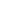Category List

# coaxial open-short circuitor

#### Product List

PRODUCT DESCRIPTION

It is an open coaxial and coaxial plunger together a coaxial components, mainly for coaxial measurement system calibration using.

Type DescriptionCoaxial Short CIRCUITOR;

Frequency Range0-3GHz;

Connection Type: N-J

Coaxial connector type

N=N-K   NJ=N-J   S=SMA-K   SJ=SMA-J

TNC=TNC-K    TNCJ=TNC-J

BNC=TNC-K   BNCJ=TNC-J   K=K2.92-K   KJ=k2.92-J   V=V2.4-K  VJ=V2.4-J     L16=L16-K    L16J=L16-J   L29=L29-K    L29J=L29-J

DATA SHEET

 Product Type Frequency Range (GHz) VSWR  (Min.) Connection  Type Characteristic impedance (Ohm) HI180COSNJJ DC-18 50 N-K 50Ω HI180COSNKK DC-18 50 N-J 50Ω HI180COSSJJ DC-18 50 N-K 50Ω HI180COSSKK DC-18 50 N-J 50Ω